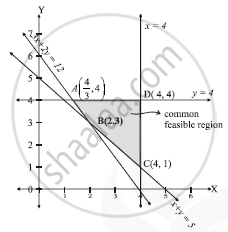# Minimize :Z=6x+4y, Subject to : 3x+2y ≥12 - Mathematics and Statistics

Minimize :Z=6x+4y

Subject to : 3x+2y ≥12

x+y ≥5

0 ≤x ≤4

0 ≤ y ≤ 4

#### Solution

3x+2y ≥12

Points : (4, 0) and (0, 6), Non origin side

x+y ≥5

Points : (5, 9) and (0, 5), Non origin side

0 ≤x ≤4

Parallel to y-axis, point (4, 0), origin side

0 ≤ y ≤ 4

Parallel to x-axis, point (0, 4), origin side

x ≥ 0, y ≥ 0
x-axis and y-axis, first quadrant only.A is the intersection of 3x+2y =12 and y= 4

x=4/3 and y=4

A(4/3, 4)

B is intersection of 3x + 2 y = 12 and x + y= 5
x=2, y=3
B(2,3)

C is the intersection of x = 4 and x + y = 5
x=4, y=1
C(4,1)

D is the intersection of x = 4 and y = 4

D ( 4, 4)

 End Points value of z=6x+4y A(4/3, 4) 8+16=24 B(2, 3) 12+12=24 C(4, 1) 24+4=28 D(4, 4) 24+16=40

Z is minimum 24 on the segment AB joining A( 4/3 ,4) and (2,3)

Concept: Graphical Method of Solving Linear Programming Problems
Is there an error in this question or solution?